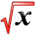# Partial DerivativesThis article is part of the MathHelp Tutoring Wiki

Function f(x,y) of two variables, there are two partial derivatives ∂f/∂x and ∂f/∂y.

∂f/∂x (a,b) = lim(h→0) f( a+h , b ) - f( a , b )
h
∂f/∂y (a,b) = lim(h→0) f( a , b+k ) - f( a , b )
k
• For ∂f/∂x , f is differentiated as a function with respect to x and y is treated as a constant.
• For ∂f/∂x , f is differentiated as a function with respect to y and x is treated as a constant.

Example 1 :

Solve for ∂f/∂x and ∂f/∂y if f(x,y) = $-x^{2}+y$.
Solution :
∂f/∂x = -2x
∂f/∂y = 1

Example 2 :

Solve for ∂g/∂x (3,2) and ∂g/∂y (3,2) if g(x,y) = xye^$x^{2}$.
Solution :
∂g/∂x = (xy)*(2xe^$x^{2}$) + ye^$x^{2}$= 2$x^{2}$ye^$x^{2}$+ ye^$x^{2}$=ye^$x^{2}$(2$x^{2}$+1)
∂g/∂x (3,2) = 2e^$3^{2}$(2$3^{2}$+1)
= 2$e^{9}$(19)
= 38$e^{9}$∂g/∂y = xe^$x^{2}$∂g/∂y (3,2) = 3$e^{9}$Example 3 :

Solve for ∂f/∂x and ∂f/∂y if f(x,y)= ln(xy) +sin(x) = ln(x) + ln(y)+ sin(x).
Solution :
∂f/∂x = $1/xy*y+cos(x)=1/x+cos(x)$∂f/∂y = $1/xy*x=1/y$Example 4 :

Find the rectangular box of least surface area that has volume 1000$in^{3}$.
Solution :
Volume = 1000 = xyz
Surface Area = 2xz + 2yz + 2xy
z = 1000/xy
S = 2x(1000/xy) + 2y(1000/xy) + 2xy
= 2000/y + 2000/x + 2xy *need to minimize this*
Solve for ∂S/∂x = 0 and ∂S/∂y = 0
∂S/∂x = -2000/$x^{2}$+ 2y = 0
∂S/∂y = -2000/$y^{2}$+ 2x = 0
y = 1000/$x^{2}$and x = 1000/$y^{2}$y($y^{3}-1000$) = 0
Therefore, y = 0 or $y^{3}$-1000 = 0
So, y = 10 and x = 10.
Using the second derivative test, S does have a local minimum at (10, 10).